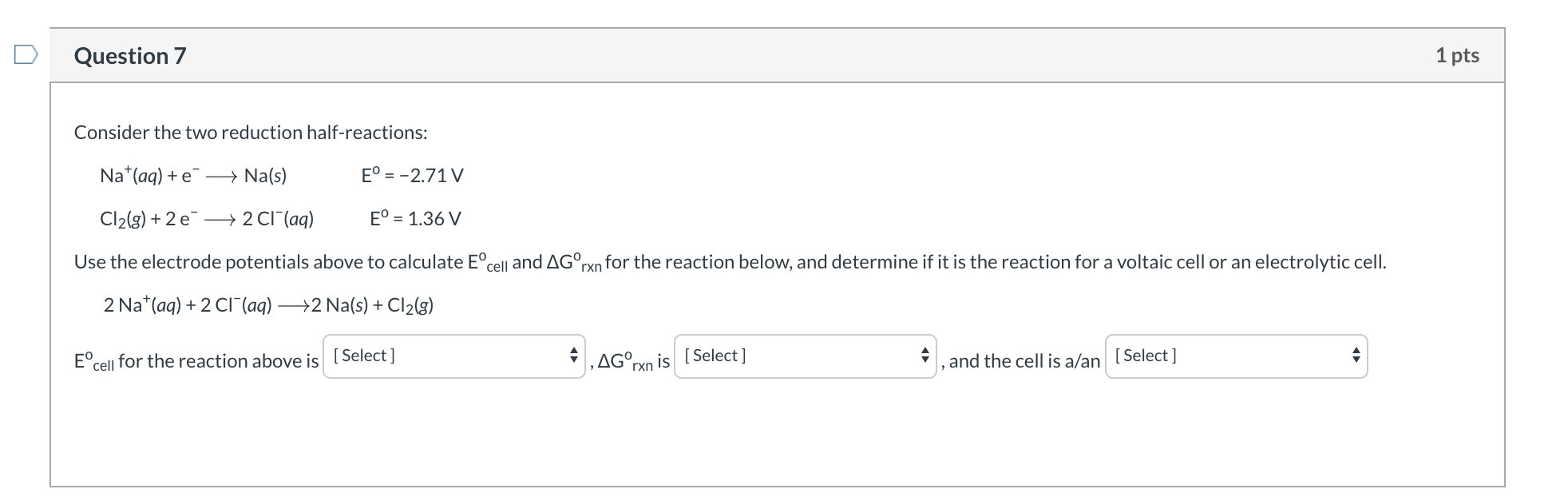# Consider the two reduction half-reactions: Na+(aq) + e− ⟶ Na(s) Eo = −2.71 V Cl2(g) + 2 e− ⟶ 2 Cl−(aq) Eo = 1.36 V Use the electrode potentials above to calculate Eocell and ∆Go Rxn for the reaction below, and determine if it is the reaction for a voltaic cell or an electrolytic cell. 2 Na+(aq) + 2 Cl−(aq) ⟶2 Na(s) + Cl2(g) Eocell for the reaction above is , ∆Go Rxn is , and the cell is a/an